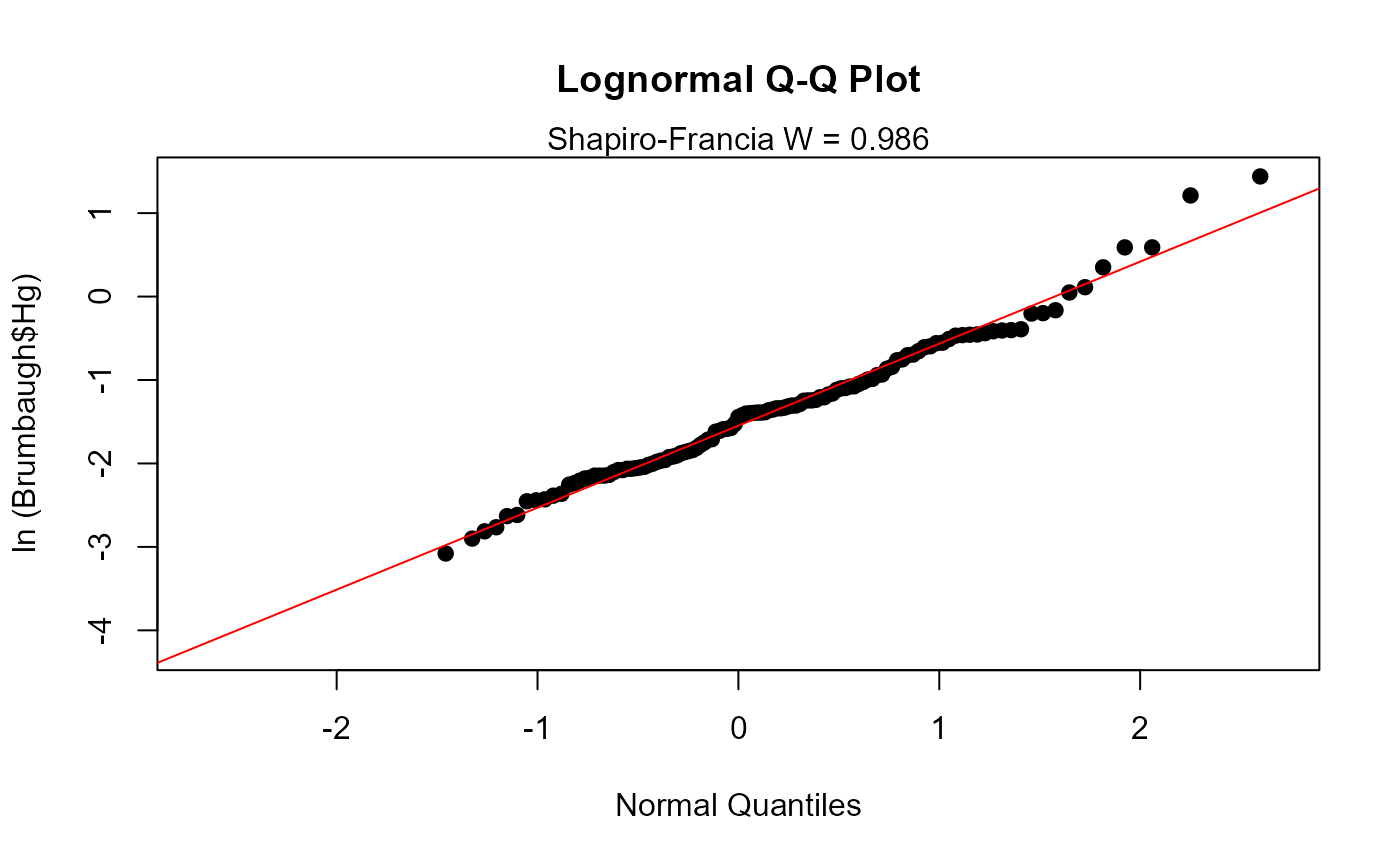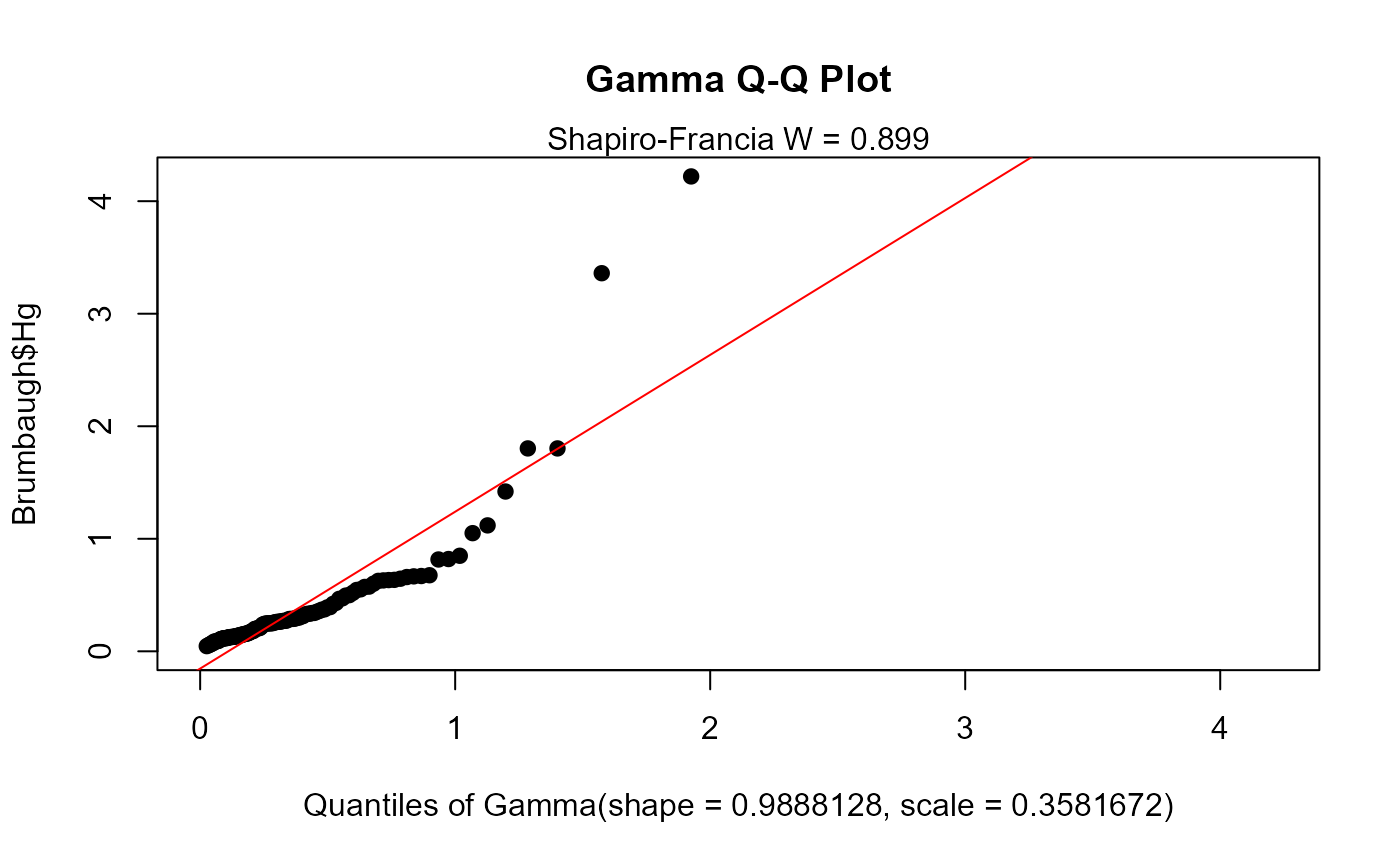Plots a quantile-quantile (Q-Q) plot of censored data versus a fitted data distribution

cenQQ(x.var, cens.var, dist = "lnorm", Yname = yname)

## Arguments

x.var

The column of x (response variable) values plus detection limits

cens.var

The column of indicators, where 1 (or TRUE) indicates a detection limit in the y.var column, and 0 (or FALSE) indicates a detected value in y.var.

dist

One of three distributional shapes to fit to your data: lognormal (lnorm), normal (norm) or gamma (gamma).

Yname

Optional – input text in quotes to be used as the variable name on the Q-Q plot. The default is the Yname name of the y.var input variable.

## Value

A single Q-Q plot of data fitted by normal, lognormal or gamma distributions with Shapiro-Francia W value printed on plot.

## Examples

# \donttest{
data(Brumbaugh)
cenQQ(Brumbaugh$Hg,Brumbaugh$HgCen)# User defined distribution
cenQQ(Brumbaugh$Hg,Brumbaugh$HgCen,dist="gamma")# }